# 18. Under conditions of constant temperature and pressure, one mol of any gas will A. never...

18. Under conditions of constant temperature and pressure, one mol of any gas will

A. never occupy the same volume
B. always react with 2 moles of gas
C. alter its volume based on the molarity
D. always occupy the same volume

19. what is the molar volume of a gas?
A. it is the molar mass of an ideal gas.
B. it is the volume of one mol of gas.
C. it is the number of grams per volume of a gas.
D. it is the number of microns per volume of gas.

18. Under conditions of constant temperature and pressure, one mole of any gas will (At STP conditions one mole of gas occupies 22.4 liters of the volume).

A. never occupy the same volume

B. always react with 2 moles of gas

C. alter its volume based on the molarity

D. always occupy the same volume

Answer) D. always occupy the same volume i.e. 22.4 liters of volume.

19. what is the molar volume of a gas? ( Molar volume of gas is defined as volume occupied by one mole of gas)

A. it is the molar mass of an ideal gas.

B. it is the volume of one mole of gas.

C. it is the number of grams per volume of a gas.

D. it is the number of microns per volume of gas.

Answer) B. it is the volume of one mole of gas.

#### Earn Coin

Coins can be redeemed for fabulous gifts.

Similar Homework Help Questions
• ### chapter question a) If 0.00954 mol neon gas at a particular temperature and pressure occupies a...

chapter question a) If 0.00954 mol neon gas at a particular temperature and pressure occupies a volume of 219 mL, what volume would 0.00784 mol neon occupy under the same conditions? WebAssign will check your answer for the correct number of significant figures. “answer in mL” b)If 2.77 g of argon gas occupies a volume of 4.29 L, what volume will 1.28 mol of argon occupy under the same conditions? WebAssign will check your answer for the correct number of...

• ### Pre-lab for Molar Volume of a Gas How much volume does a gas occupy under standard conditions of temperature (O°C o...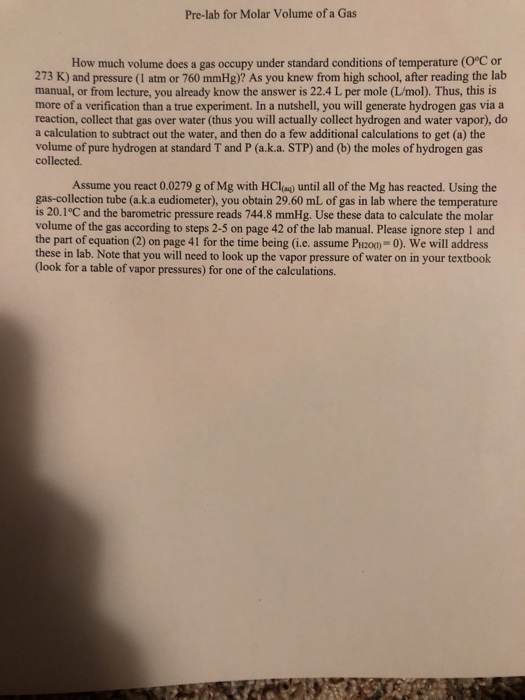Pre-lab for Molar Volume of a Gas How much volume does a gas occupy under standard conditions of temperature (O°C or 273 K) and pressure (1 atm or 760 mmHg)? As you knew from high school, after reading the lab manual, or from lecture, you already know the answer is 22.4 L per mole (L/mol). Thus, this is more of a verification than a true experiment. In a nutshell, you will generate hydrogen gas via a reaction, collect that gas...

• ### Under constant-volume conditions, 3100 J of heat is added to 1.9 moles of an ideal gas....

Under constant-volume conditions, 3100 J of heat is added to 1.9 moles of an ideal gas. As a result, the temperature of the gas increases by 78.5 K. How much heat would be required to cause the same temperature change under constant-pressure conditions? Do not assume anything about whether the gas is monatomic, diatomic, etc.

• ### Under constant-volume conditions, 4100 J of heat is added to 1.5 moles of an ideal gas....

Under constant-volume conditions, 4100 J of heat is added to 1.5 moles of an ideal gas. As a result, the temperature of the gas increases by 132 K. How much heat would be required to cause the same temperature change under constant-pressure conditions? Do not assume anything about whether the gas is monatomic, diatomic, etc.

• ### At standard temperature and pressure (0 ∘C and 1.00 atm ), 1.00 mol of an ideal...

At standard temperature and pressure (0 ∘C and 1.00 atm ), 1.00 mol of an ideal gas occupies a volume of 22.4 L. What volume would the same amount of gas occupy at the same pressure and 75 ∘C ?

• ### Please answer & explain 26-30 A gas sample is held at constant pressure. The gas occupies...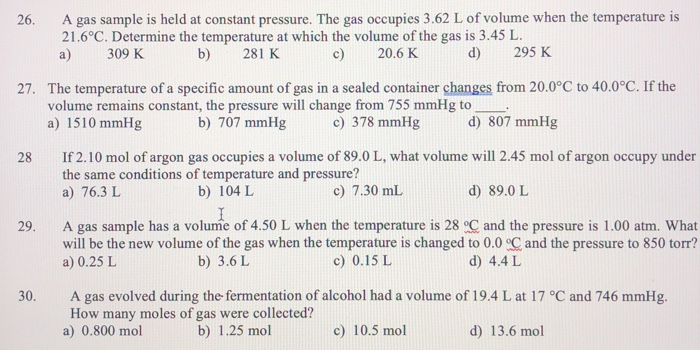Please answer & explain 26-30 A gas sample is held at constant pressure. The gas occupies 3.62 L of volume when the temperature is 21.6°C. Determine the temperature at which the volume of the gas is 3.45 L 26 d) 295 K 309 K b) 281 K c) 20.6 K a) 27. The temperature of a specific amount of gas in a sealed container changes from 20.0°C to 40.0°C. If the volume remains constant, the pressure will change from 755...

• ### Experiment is done at the following conditions. Temperature of boiling water (°C) 99.2 Atmoshperic Pressure (torr)...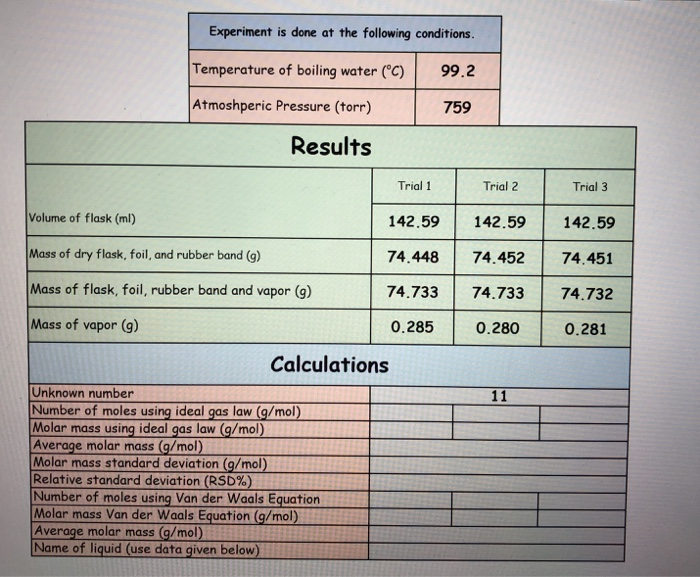Experiment is done at the following conditions. Temperature of boiling water (°C) 99.2 Atmoshperic Pressure (torr) 759 Results Trial 1 Trial 2 Trial 3 Volume of flask (ml) 142.59 142.59 142.59 Mass of dry flask, foil, and rubber band (g) 74.448 74.733 74.45274.451 74.733 74.732 Mass of flask, foil, rubber band and vapor (9) Mass of vapor (9) 0.285 0.2800.281 Calculations Unknown number Number of moles using ideal gas law (g/mol) Molar mass using ideal gas law (g/mol) Average molar...

• ### Under conditions of constant temperature and volume, how many additional moles of gas would have to...Under conditions of constant temperature and volume, how many additional moles of gas would have to be added to a flask containing 1.96 moles of gas at 25.0 C and 1.00 atm pressure in order to increase the pressure to 2.3 atm?

• ### Calculate and use the molar volume of a gas under ndard temperature and pressure conditions Question...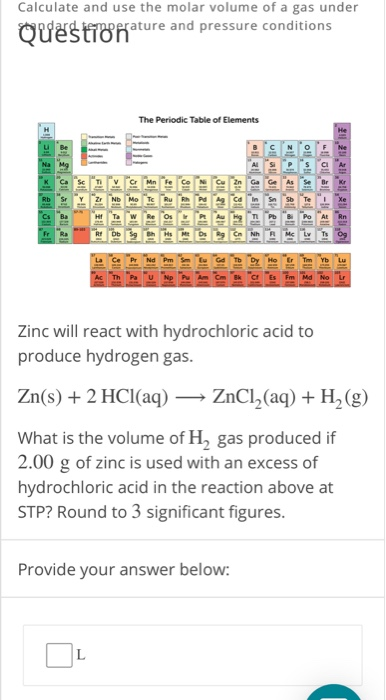Calculate and use the molar volume of a gas under ndard temperature and pressure conditions Question The Periodic Table of Elements He Rn Fr Ho Er Tm Md No Zinc will react with hydrochloric acid to produce hydrogen gas. Zn(s)+2 HCl(aq) ZnCl2(aq)H2 (g) What is the volume of H2 gas produced if 2.00 g of zinc is used with an excess of hydrochloric acid in the reaction above at STP? Round to 3 significant figures. Provide your answer below: Oal-...

• ### Problem 1. Which statement below is true (choose only one): (a) A mol of any gas...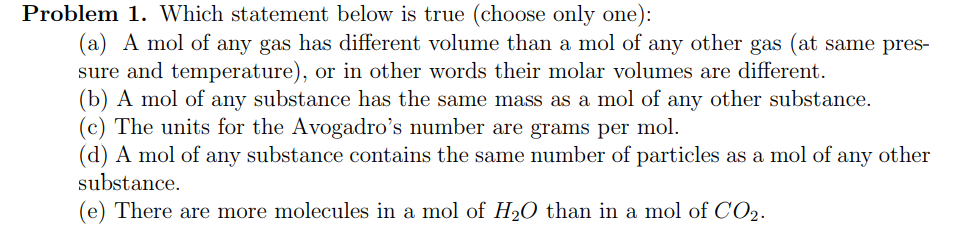Problem 1. Which statement below is true (choose only one): (a) A mol of any gas has different volume than a mol of any other gas (at same pres- sure and temperature), or in other words their molar volumes are different. (b) A mol of any substance has the same mass as a mol of any other substance. c) The units for the Avogadro's number are grams per mol (d) A mol of any substance contains the same number of...